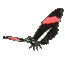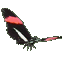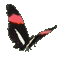Trigonometry Ratios for Angles based on 0o,30o,45o,60o,90o - Game Tips:

- The four quadrants of a Math graph resemble the 4 regions of a map.
Starting from the centre, the North-East region is quadrant 1. The North-West region is quadrant 2.
The South-West region is quadrant 3, and the South-East region is quadrant 4.

- Positive angles in standard position at the origin (0,0) are formed with initial arm always pointing East
and rotating the second arm of the angle counterclockwise to a terminal position.
Angles that rotate clockwise are negative angles in Mathematics.

- When angle DAB is in standard position at a graph's origin (0,0) then sin A equals (y/r),
cos A equals (x/r), and tan A = (y/x).
- When r = 1 then the primary Trig ratios simplify to sin A = y, cos A = x, and tan A = (y/x).

- Notice 30,60,90 right triangles have sides in the special ratio 0.5 / 0.866 / 1.0 approx.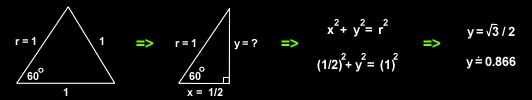- Notice 45,45,90 right triangles have sides in the special ratio 0.707 / 0.707 / 1.0 approx.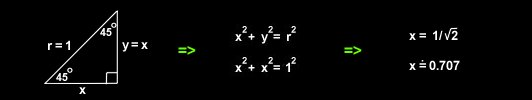- When the terminal arm goes from (0,0) to (0,1) then the angle is 90o.
- When the terminal arm goes from (0,0) to (-1,0) then the angle is 180o.
- When the terminal arm goes from (0,0) to (0,-1) then the angle is 270o.
- When the terminal arm goes from (0,0) to (1,0) then the angle is 0o or 360o.

- The special angles based on 60o, are 120o, 240o, and 300o.
- When the terminal arm goes from (0,0) to (0.5,0.866) then the angle is 60o.
- When the terminal arm goes from (0,0) to (-0.5,0.866) then the angle is 120o.
- When the terminal arm goes from (0,0) to (-0.5,-0.866) then the angle is 240o.
- When the terminal arm goes from (0,0) to (0.5,-0.866) then the angle is 300o.

- The special angles based on 30o, are 150o, 210o, and 330o.
- When the terminal arm goes from (0,0) to (0.866,0.5) then the angle is 30o.
- When the terminal arm goes from (0,0) to (-0.866,0.5) then the angle is 150o.
- When the terminal arm goes from (0,0) to (-0.866,-0.5) then the angle is 210o.
- When the terminal arm goes from (0,0) to (0.866,-0.5) then the angle is 330o.

- The special angles based on 45o, are 135o, 225o, and 315o.
- When the terminal arm goes from (0,0) to (0.707,0.707) then the angle is 45o.
- When the terminal arm goes from (0,0) to (-0.707,0.707) then the angle is 135o.
- When the terminal arm goes from (0,0) to (-0.707,-0.707) then the angle is 225o.
- When the terminal arm goes from (0,0) to (0.707,-0.707) then the angle is 315o.

- The CAST Rule is a memory aid to help remember that
the Cosine ratio is positive in the 4th quadrant,
All the primary Trig ratios are positive in the first quadrant,
the Sine ratio is positive in the 2nd quadrant, and
the Tangent ratio is positive in the 3rd quadrant.

- Your Game Score is reduced by the number of butterfly hits.

- To slow the game speed repeat tap/click on the word Slider.
- To increase the game speed repeat tap/click on the word Math.
- Speed can also be adjusted with a keyboard's - and + keys.

- Refresh/Reload the web page to restart the game.